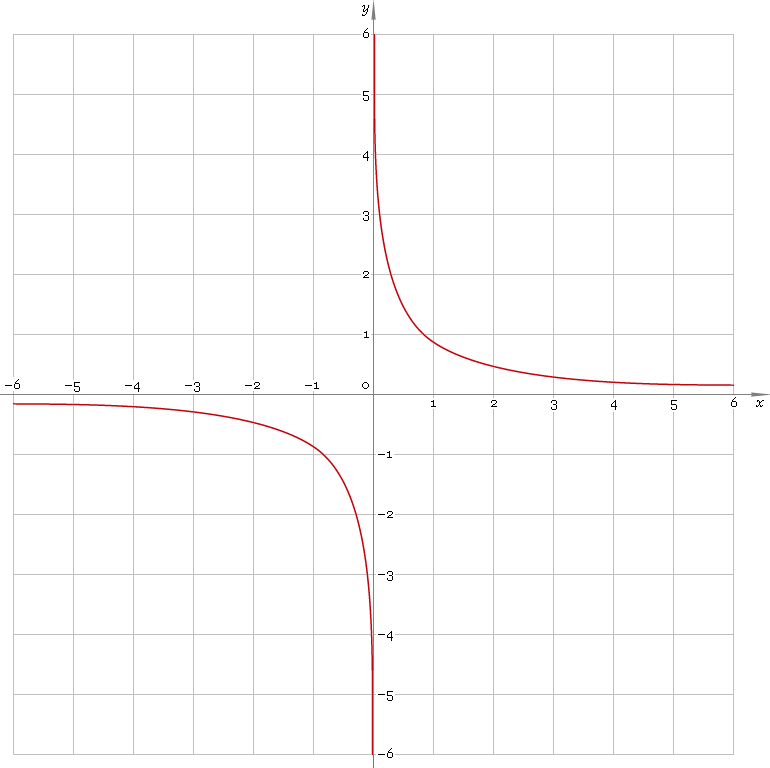The Art of Mathematics

# arcsch — arc-hyperbolic cosecant function

## 1. Definition

Arc-hyperbolic cosecant is inverse of hyperbolic cosecant function.

arcschxcschinvx

With the help of natural logarithm it can be represented as:

arcschx ≡ ln[1/x + √(1/x2 + 1)]

## 2. Graph

Arc-hyperbolic cosecant is antisymmetric function defined everywhere on real axis, except its singular point 0 — so its domain is (−∞, 0)∪(0, +∞). Function graph is depicted below — fig. 1.Fig. 1. Graph of the arc-hyperbolic cosecant function y = arcschx.

Function codomain is all real axis, except 0: (−∞, 0)∪(0, +∞).

## 3. Identities

Property of antisymmetry:

arcsch−x = −arcschx

Reciprocal argument:

arcsch(1/x) = arsinhx

Sum and difference:

arcschx + arcschy = arcsch{xy / [x√(1 + 1 /x2) + y√(1 + 1 /y2)]}
arcschx − arcschy = arcsch{xy / [y√(1 + 1 /y2) − x√(1 + 1 /x2)]}

## 4. Derivative and indefinite integral

Arc-hyperbolic cosecant derivative:

arcsch′x = −1 /[|x| √(1 + x2)]

Indefinite integral of the arc-hyperbolic cosecant:

∫ arcschx dx = x arcschx + ln[x + √(1 + x2)] + C

where C is an arbitrary constant.

## 5. How to use

To calculate arc-hyperbolic cosecant of the number:

``arcsch(−1);``

To get arc-hyperbolic cosecant of the complex number:

``arcsch(−1−i);``

To get arc-hyperbolic cosecant of the current result:

``arcsch(rslt);``

To get arc-hyperbolic cosecant of the number z in calculator memory:

``arcsch(mem[z]);``

## 6. Support

Arc-hyperbolic cosecant of the real argument is supported in free version of the Librow calculator.

Arc-hyperbolic cosecant of the complex argument is supported in professional version of the Librow calculator.